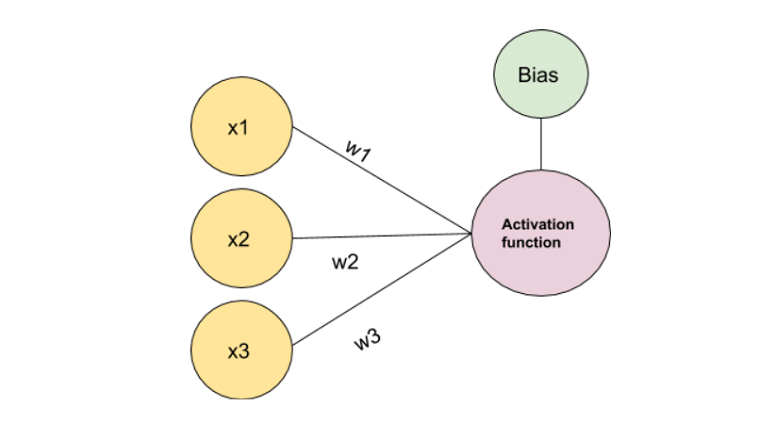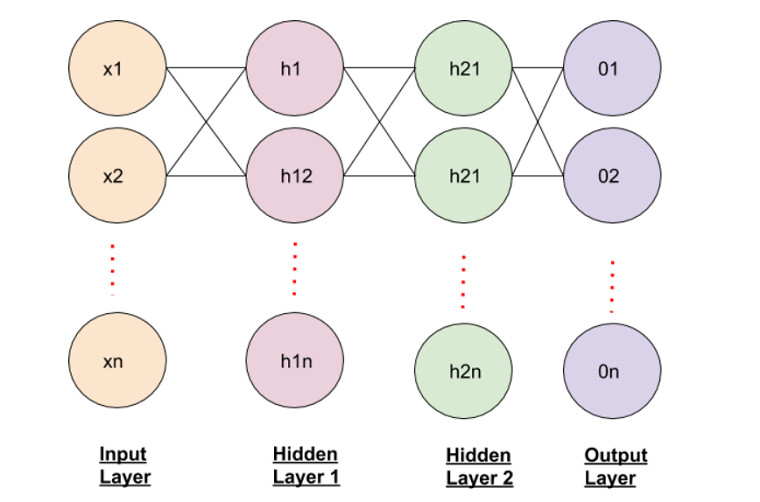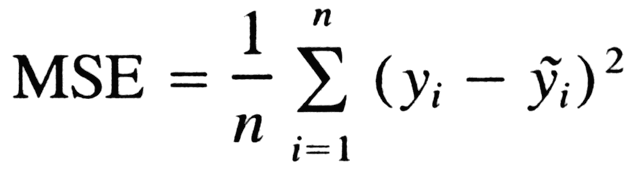• Category
• >Deep Learning

# Introduction to Neural Networks and Deep Learning

• Rohit Dwivedi
• May 16, 2020
• Updated on: Jul 02, 2021## Introduction

Here is something that would make you surprised. Do you think Neural networks are too complex jargon? No, it’s not, it is simpler than people think. In this blog, my main objective of mine is to make you familiar with Deep Learning and Neural Networks.

In this blog, I would be discussing how neural networks work. What are the different segments in the Neural Network? How is input is given to the neural network and how the output is computed?

Let's start with a basic building block of neural networks that is a “Neuron” which is also called a perceptron. It takes inputs, does calculation and mathematics inside and gives out an output.

The below image is an example of a 3 input neuron that are  (x1,x2,x3) and corresponding are the weights (w1,w2,w3). After the input is fed into the neuron there is an “Aggregation Function” & “Activation Function” that comes into play.The structure of Neuron

• Here first inputs are multiplied by weights.

• Addition of all weighted inputs with a bias b.

• The sum is passed through an activation function.

(Must read: Perceptron Model in Machine Learning)

### Aggregation Function

1. Inside a neuron, the weights and inputs to the neurons make interaction and then are aggregated into a single value.

2. The way by which we calculate input from other earlier neurons is called the “Aggregation function”.

3. There are many different aggregation functions that are used. Few are listed below:

• Sum of Product

• Product of Sum

• Division of Sum

• Division of Product

### Activation Function

1. Its main function is to activate the neuron for the required outputs.

2. It transforms linear input to non-linear so as to get good results.

3. It is also used to normalize the output between the range of (1 to -1).

4. Like aggregation functions you can define any function as an activation function.

5. Some of the most used Activation functions is stated below:

• Sigmoid
• Softmax
• Relu
• Tanh
• Leaky Relu

## Neural Network

Neural networks depict the human brain behaviour that allows computer programs to identify patterns and resolve problems in the field of AI, machine learning and deep learning.

A neuron in the neural network is a mathematical function that accumulates and categorizes information according to a neural architecture where each neural network incorporates different layers of interconnected nodes. These nodes are perceptron and are identical to multiple linear regression.The basic structure of a Neural Network

The above image shows the basic structure of a neural network that has inputs that are x1,x2 and so on. These inputs are connected to two different hidden layers and continued and at last, there is an output layer that is y1,y2 and so on.

### Feed Forward-

• It is a connection from inputs towards the output.

• There is no connection that is connected to backwards.

### Consists of different layers-

• The current layers input is the previous layer output.

• There are no intra-layer connections that are present.

### Different layers in Neural Networks

1. Input Layer: Its function is to define the input vector. These usually form an input layer and there is only one layer that is present.

2. Hidden Layer:  These layers constitute the intermediary node that divides the layer into boundaries. These form the hidden layers. We can model an arbitrary input-output relation if there are many hidden nodes.

3. Output Layer: This layer is responsible for the output of the neural network. If there are two different classes there is only one output node.

#### Importance of hidden layers

• The first hidden layer extracts features.

• The second hidden layer extracts features of features.

• Output layers give the desired output.

### Here,

• The weight gives the matrix.

• Vectors are formed from the output of the previous layer.

• The activation function is applied pointwise to the weighted times of the input.

### What needs to be taken care of while designing a neural network?

• You need to check about the layers you want to use. (Dependent upon the data)

• You need to take care of the neuron required in each layer.

• Output and input always depends upon the problem statement but you can always choose or make a choice between neurons and hidden layers.

### Training a neural network

For given xi and yi

1. Calculate fw(xi) as an estimate of yi for all the samples.

2. Calculate the loss.

3. Make changes in w as to lower down the loss as little as possible.

4. Repeat the steps.

### Loss function

Loss function also known as cost function tells you about the performance of your model for making the desired predictions. It calculates the error for each training whereas cost functions computes the average loss function of all training samples. Enhance your learning with types of loss functions in neural networks.

Assume there are total N data points in the data. We want to compute loss for all N data points that are present in the data. Then the loss can be computed using the below formula.Loss function

(Similar read: A cost function in machine learning)

### How to lower the down loss?

If the loss will be less, the model would be able to generalize. But why is it so? This is because if you will decrease the error between the predicted value and the actual value, that means the model is performing well.

How to lower down the loss lets us understand an algorithm known as Gradient Descent.

It is called the optimization algorithm that is used widely as a loss function. It is a method to optimize neural networks. It is used to compute the minimum values for a respective function. It is also termed as Back Propagation.

### Learning Rate

It is defined as the weights that are changed in different epochs (training of each layer is called as one epoch)  are called Learning rate or step size. The learning rate is represented by a symbol called a greek letter (n).

While the training is taking place the backpropagation computes the errors that are directly responsible for the weights of the node. The weights are ascended by step size instead of changing the whole weight.

That meant a step size of 0.1 that is the default value would signify that weights in the networks are updated 0.1* (computed weight error) or 10% of the computed weight error for each period of time the weights are changed.

(Suggested blog: Keras tutorial: A Neural Network Library in Deep Learning)

## Conclusion

I would conclude this blog by stating that if the amount of the data increases the computation power also increases and that is where neural networks give you a good performance.

In this blog, I have taken you through the concept of the neuron, neural network, aggregation functions, activation functions, different layers in the neural network, how to train a neural network, the role of optimization, and learning rate.

I hope you might have got a basic idea behind neural networks. In the end, you can learn applications of deep learning.

•#### rajatktiwari1997

May 16, 2020

Well Explained .

•#### 360digitmgsk

Oct 31, 2020

•